x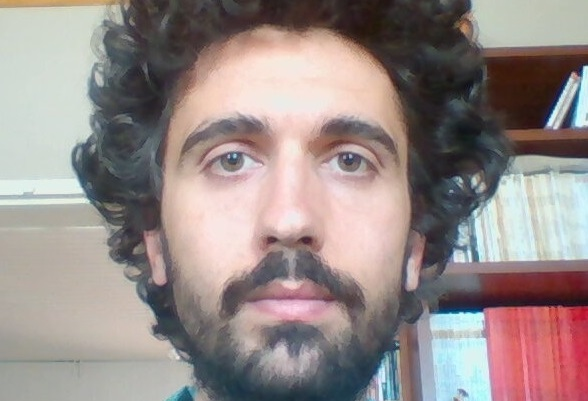Postdoctoral Fellow

Octave Curmi is a postdoc working in topology of singularities and
geometry. He studies mainly the so-called smoothings of singularities of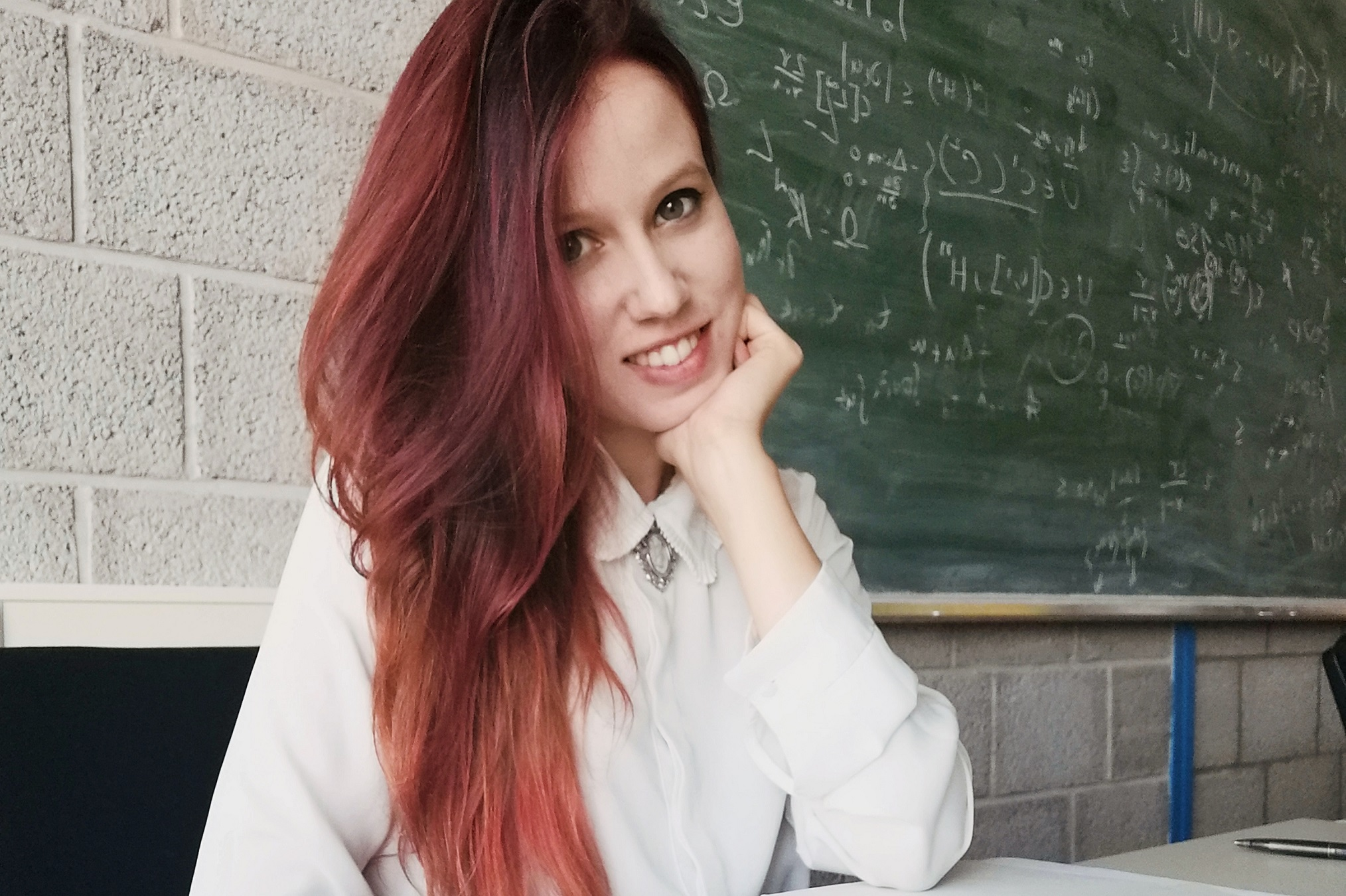Postdoctoral Fellow

Danica Basarić is a postdoc working in fluid dynamics.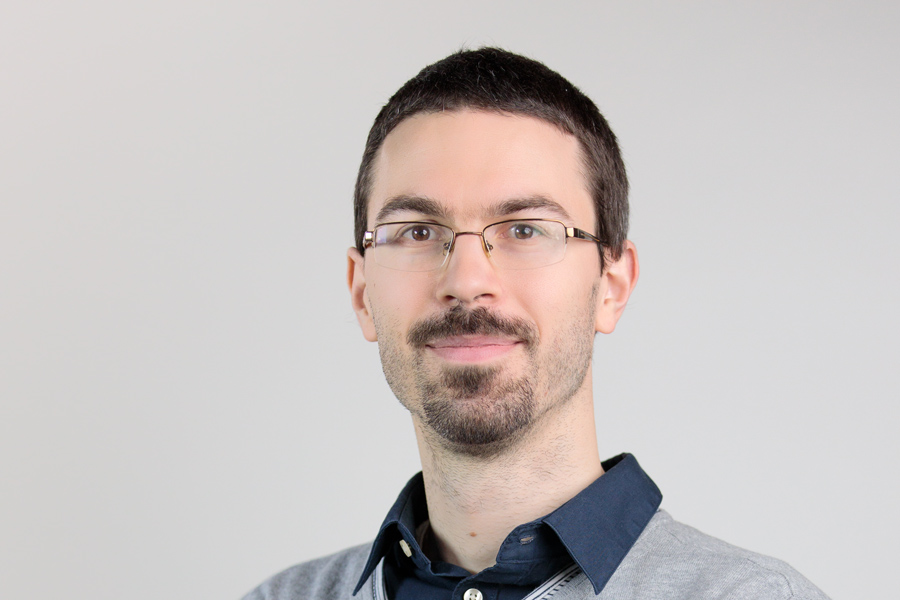Researcher

Dávid Kunszenti-Kovács studies various questions within graph limit theory.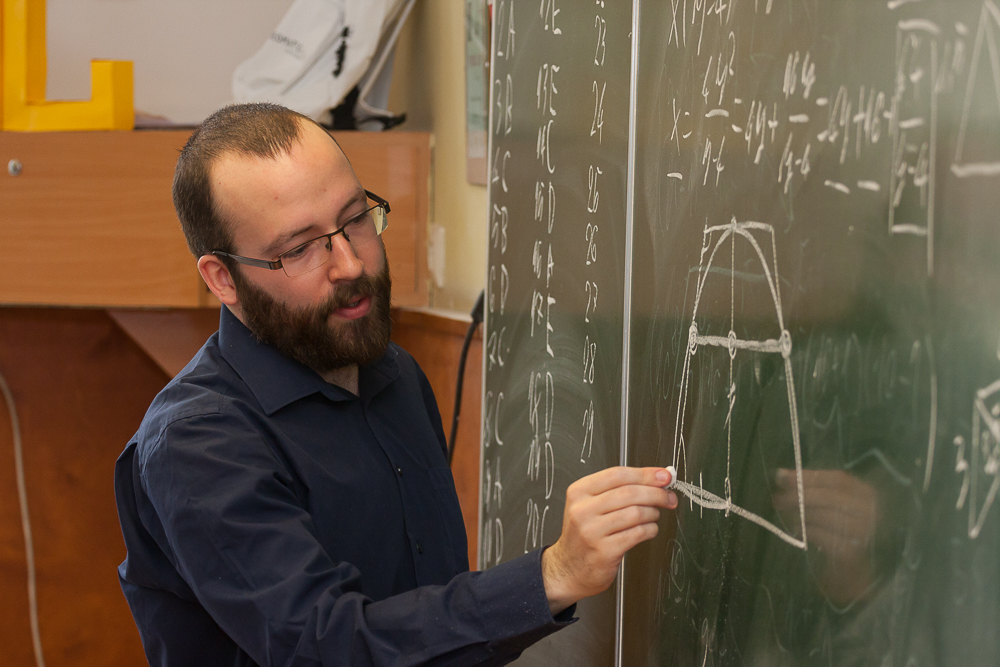PhD Student
Gábor Damásdi is a Hungarian doctoral student at Eötvös Loránd University. His research interest lies in combinatorial and discrete geometry and computer science.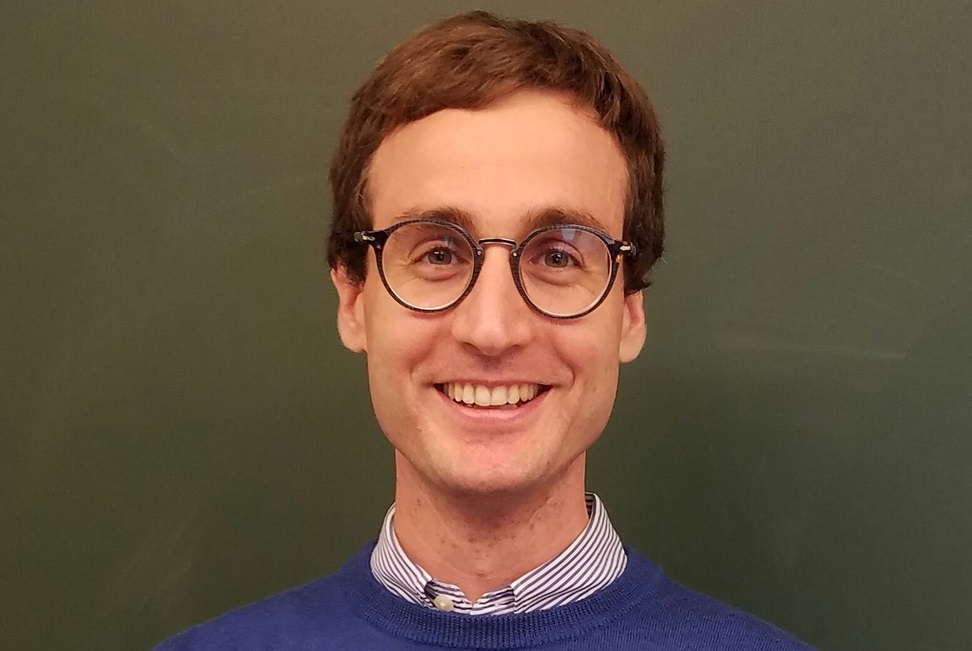Postdoctoral Fellow

Marco Marengon is a postdoc working in topology.

2021.12.01. - 2021.12.01.
Rényi Institute, Main Lecture Hall
This talk aims at providing an introduction to some concepts from singularity theory, and in particular singularities of complex surfaces, and their relation to lowdimensional topology.
2021.11.03. - 2021.11.03.
Rényi Institute, Main Lecture Hall
In the first part of the talk we will provide a fairly complete proofs of the 2-squares Theorem (Fermat), the 4-squares theorem (Lagrange) and of the 3-squares theorem (Gauss/Legendre). In the second part, we will describe the shape of the set of such representations.
2021.10.20. - 2021.10.20.
Rényi Institute, Main Lecture Hall
We survey some basic results in geometric graph theory and highlight some open problems whose solution may be within reach.
Subscribe to 2021 Fall